# CFD Analysis on an Elliptical Chamber Muffler of a C.I. Engine

CFD Analysis on an Elliptical Chamber Muffler of a C.I. Engine

Sweta Baruah Sushovan Chatterjee

Department of Engineering Design, IIT Madras, India

Department of Mechanical Engineering, Cooch Behar Government Engineering College, West Bengal 736170, India

Corresponding Author Email:
sushovan.chatterjee@gmail.com
Page:
613-619
|
DOI:
https://doi.org/10.18280/ijht.370232
11 May 2018
|
Accepted:
2 June 2019
|
Published:
30 June 2019
| Citation

OPEN ACCESS

Abstract:

Highest pressure and temperature are developed inside the combustion chamber of Compressrion Ignition (C.I.) engine. As the outlet of the exhaust system is the atmosphere, there might be adverse pressure gradient in the exhaust system which can be analysed for the purpose of attenuation of pressure wave in case of conventional silencer system. This hot exhaust gas coming out through the silencer pipes in the exhuast system of automobiles can be a scope of study for Computational Fluid Dynamic (CFD) simulation. In this work, an elliptical chamber muffler model of a MAHINDRA C.I. engine is studied based on CFD analysis of the exhaust gas flow through the muffler chamber. Two designs for the aforementioned muffler are analyzed one of which consists of perforated inlet, outlet and central pipes which, if implemented in actual practice could bring about better and improved sound attenuation. Transmission loss is calculated for both the muffler models based on pressure distribution obtained from CFD analysis results. Comparative study of the two muffler models, one without the presence of any perforation and the other after incorporation of perforation, is carried out in ANSYS FLUENT 14.5.

Keywords:

CFD simulation, muffler acoustics, transmission loss, turbulence modelling

1. Introduction

Noise generated by automobile exhaust is one of the key factors of environmental pollution posing as a hazard to human health. As such, maintaining a healthy environment necessitates reducing vehicle exhaust noise to a bare minimum. An engine muffler or silencer plays the pivotal role of minimizing this noise generated due to flow of exhaust gas at high pressure and temperature by either destructive interference of the sound waves or absorbing these waves in sound absorbent material.

The parameters for determining the performance of muffler are Noise reduction, Transmission loss and Insertion loss. Noise reduction is basically the difference in sound pressure levels on the source side and receiver side. Transmission loss can be defined as the difference between the sound power incident at the inlet of the muffler and that transmitted to the exit whereas insertion loss can be defined as the difference between acoustic power radiated without and with a muffler.

Munjal  through his work brought about the inception of muffler classification based on their working principle. He stated primarily two types of muffler, viz., Passive mufflers, also known as reflective or reactive mufflers, which works on the principle of impedance mismatch and Dissipative mufflers which works on the principle of energy conversion from acoustic to heat by means of highly porous linings.

Acoustic behavior of elliptical chamber muffler was studied taking into account the effect of chamber length, location of inlet and outlet ports and eccentricity of the ellipse . Analysis was performed on expansion chambers and reversing chambers with inlet and outlet ports located on the major axis of the ellipse. Results obtained were seen to have a good agreement with those obtained from Finite Element Method and experimental study.

Gerges et al.  stated that the transfer matrix method could be used for prediction of transmission loss of mufflers in the low frequency range. It is possible to use this method for designing a muffler prototype that can reduce the noise produced at firing frequency of the engine neglecting effects of higher order modes. Several muffler configurations were measured experimentally for transmission loss and the results were found to be in good agreement with those obtained numerically from transfer matrix method.

Sohei et al.  derived the four pole parameters of an elliptical chamber muffler with a perforated pipe by solving the governing equations of these four pole parameters. A detailed examination of a particular parameter without considering the effect of mean flow velocity yielded similar results as that of experimental verification thus validating the same.

Caradonna et al.  combined CFD method with acoustic generation and propagation theory to put forward an innovative approach for aero-acoustic prediction of flow noise inside complex exhaust mufflers. Simulation of flow generated noise was achieved by two approaches using combination of aerodynamics and acoustics using convective scalar wave equations. Results obtained were verified using several turbulent models and silencers of increased complexity.

Kang et al.  proposed a three-dimensional FEM model to compute acoustic attenuation performance of straight through perforated duct mufflers without taking into account any area discontinuity which the flow may witness in real practice. Acoustic attenuation performance was predicted by measurement of transmission loss. For low Mach number predicted results were in good agreement with experimental results whereas for high Mach number agreement existed only in the low and mid frequency range and not much in the high frequency range.

Bhangale et al.  presented a plane wave transfer matrix model for designing muffler prototype in minimum time, calculating their transmission loss and then comparing the results with experimental values. The use of 3D techniques like FEM and BEM requires a lot of time as well as expertise for use.

Wankhade et al.  presents an effective elliptical silencing model which is simple in construction and provides efficient sound attenuation. An optimal design scheme is proposed for improved muffler performance using FEM and Taguchi method. Transmission loss was found to increase in the elliptical muffler model having an extra divided inlet tube along with extended inlet and outlet.

Chatterjee  has also performed CFD simulation study on various absorptive and reactive muffler models.

Baruah and Chatterjee  have reported on structural analysis of the similar muffler geometry under static and dynamic loading conditions in order to monitor the response spectra by harmonic analysis. There, the static and dynamic structural analysis of a proposed elliptical chamber muffler design has been performed to obtain stress, strain and defomation contours. Incorporation of perforation is seen to give significant sound attenuation with reduced stress and strain.

The scope of current work is restricted to the study of results of CFD simulations of the exhaust hot gas flow from exhaust port of an automotive engine to the atmosphere through the designed perforated and non-perforated models of silencer pipe. Present work deals with a numerically study on an elliptical chamber muffler of a C.I. engine using ANSYS FLUENT CFD coding.

2. Problem Statement and Boundary Condition

2.1 Problem statement

For the purpose of analysis, a three-chambered elliptical chamber muffler having a single inlet and single outlet is modeled. The dimensions of the muffler are in accordance with the actual dimensions of a MAHINDRA MAXIMO PLUS 2-cylinder 4-stroke CI engine. A previously existing muffler model with inlet and outlet pipes and baffle plates is created. The design proposed in this present work involves perforated pipes and the objective is to study the flow stress, sound attenuation and vibration characteristics of the muffler under the action of flow of high temperature and pressure exhaust gases.

2.2 Boundary condition

For computational analysis in FLUENT velocity-inlet boundary condition is applied at the inlet and pressure-outlet boundary condition with ambient condition of pressure and temperature is applied at the outlet of the muffler.

 Inlet: Outlet: Pressure = 290000 Pa Pressure = 101325 Pa Temperature = 850 K Temperature = 300 K Velocity = 110 m/s
3. Methodology

Apart from the governing equations of continuity, momentum and energy, Standard k-ε turbulence model is used for modeling the turbulent exhaust gas flow. It incorporates one transport equation variable as turbulent kinetic energy "k" and the other as turbulent dissipation rate "ε". The first variable "k" determines the energy in turbulence whereas the second variable determines the scale of turbulence in either length scale or time scale.

The turbulence kinetic energy "k" and its rate of dissipation "ε" are obtained from the following transport equations :

$\frac{\delta}{\delta t}(\rho k)+\frac{\delta}{\delta x_{i}}\left(\rho k u_{i}\right)=\frac{\delta}{\delta x_{j}}\left[\left(\mu+\frac{\mu_{i}}{\sigma_{k}}\right) \frac{\delta k}{\delta x_{j}}\right]+G_{k}+G_{b}-\rho \varepsilon-Y_{M}+S_{k}$ (1)

And

$\frac{\delta}{\delta t}(\rho \varepsilon)+\frac{\delta}{\delta x_{i}}\left(\rho \varepsilon u_{i}\right)=\frac{\delta}{\delta x_{j}}\left[\left(\mu+\frac{\mu_{t}}{\sigma_{\varepsilon}}\right) \frac{\delta \varepsilon}{\delta x_{j}}\right]+C_{1 \varepsilon} \frac{\varepsilon}{k}\left(G_{k}+G_{3 \varepsilon} G_{b}\right)-\\ C_{2 \varepsilon \rho} \frac{\varepsilon^{2}}{k}+S_{\varepsilon}$(2)

where,

Gk denotes the generation of the turbulence kinetic energy due to mean velocity gradients and is defined as $G_{k}=-\rho \overline{u_{\imath}^{\prime} u_{\jmath}^{\prime}} \frac{\partial u_{j}}{\partial x_{i}}$. Gk can be calculated using the Boussinesq hypothesis as $G_{k}=\mu_{t} S^{2}$ where S is the modulus of the mean rate of strain tensor defined as S = $\sqrt{2 S_{i j} S_{i j}}$.

$G_{b}$ denotes the generation of turbulence kinetic energy due to buoyancy and can be defined as $G_{b}=\cdot \beta g_{i} \frac{\mu_{t}}{\operatorname{Pr}_{t}} \frac{\partial T}{\partial x_{i}}$ where $P r_{t}$ is the turbulent Prandtl number for energy and $g_{i}$ is the component of the gravitational vector in the ith direction. The default value of $P_{r_{t}}$ is 0.85 for standard and realizable k-ε models. The co-efficient of thermal expansion $\beta$ is defined as $\beta=\frac{1}{\rho}\left(\frac{\partial \rho}{\partial T}\right)_{P}$ which finally gives the value of $G_{b}$ as $G_{b}=-g_{i} \frac{\mu_{t}}{\rho \cdot p r_{t}} \frac{\partial P}{\partial x_{i}}$.

$Y_{M}$ represents the contribution of the fluctuating dilatation in compressible turbulence to the overall dissipation rate. For high Mach number flows, compressibility affects turbulence through dilatation dissipation depicted by $Y_{M}$ in the k-equation and modeled according to Sarkar  as $Y_{M}=2 \cdot \rho \cdot \varepsilon \cdot M_{t}^{2}$ where $M_{t}$ is the turbulent Mach number defined as $M_{t}= \sqrt{K / c^{2}}$ where c = $\sqrt{\gamma R T}$ is the velocity of sound.

$C_{1 \varepsilon}$, $C_{2 \varepsilon}$, $C_{3 \varepsilon}$ are constants.

$\sigma_{k}$ and $\sigma_{\varepsilon}$ are the turbulent Prandtl numbers for k and $\varepsilon$ respectively

$S_{k}$ and $S_{\varepsilon}$ are user-defined source terms

The turbulent (or eddy) viscosity " $\mu_{t}$" is computed by combining k and $\varepsilon$ as follows:

${{\mu }_{t}}=\rho {{C}_{\mu }}\frac{{{k}^{2}}}{\varepsilon }$ (3)

where $C_{\mu}$ is constant.

The model constants $C_{1 \varepsilon}$, $C_{2 \varepsilon}$, $C_{\mu}$, $\sigma_{k}$ and $\sigma_{\varepsilon}$ have following default values :

$C_{1 \varepsilon}=1.44$, $C_{2 \varepsilon}=1.92$, $C_{\mu}=0.09$, $\sigma_{k}=1.0$ and $\sigma_{\varepsilon}$ = 1.3

4. Geometric Modeling

Geometric model for the 4-stroke 2-cylinder CI engine has been constructed on the basis of dimensions obtained from a MAHINDRA MAXIMO PLUS engine by manual measurement. The modeling has been carried out in CATIA V5 software and is illustrated in Figure 1. Figure 2 shows the meshed model for the elliptical chamber muffler wherein the geometric irregularity of an elliptical model is accounted for by using reduced element size or fine triangular mesh.

## 1.png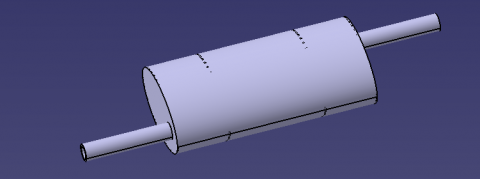Figure 1. 3-D CATIA model of the muffler

## 2.pngFigure 2. 3D meshed model of the muffler

## 3.png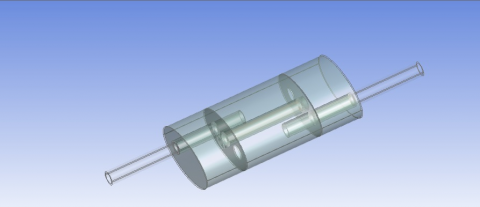Figure 3. Fluid domain for non-perforated elliptical chamber muffler model for CFD analysis

## 4.pngFigure 4. Fluid domain for perforated elliptical chamber muffler model for CFD analysis

Figure 3 and 4 illustrates the fluid domain for CFD analysis in ANSYS FLUENT for the non-perforated and perforated model respectively. The fluid flowing is assumed to be air.

The material for muffler construction is structural steel due to its high strength and corrosion resistant properties.

5. Results and Discussion

5.1 Results

CFD analysis for both the muffler models has been performed in ANSYS FLUENT 14.5 to obtain contours of pressure, velocity, turbulence and acoustic power levels.

The results obtained from CFD analysis are demonstrated below.

## 5.png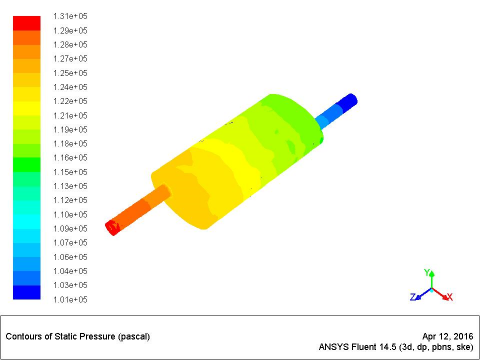Figure 5. Static pressure contour for non-perforated elliptical chamber muffler

## 6.png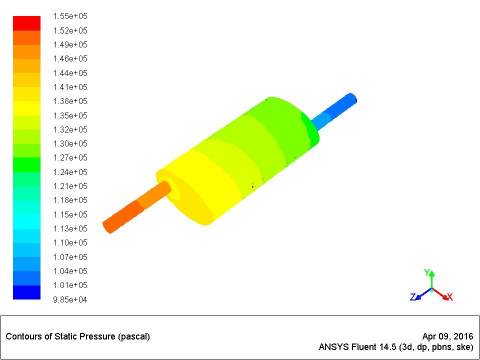Figure 6. Static pressure contour for perforated elliptical chamber muffler

Figure 5 and 6 shows the static pressure contour for the non-perforated and perforated elliptical chamber muffler model with a maximum value of 1.31×105 Pa and 1.55×105 Pa respectively at the inlet to the muffler.

## 7.png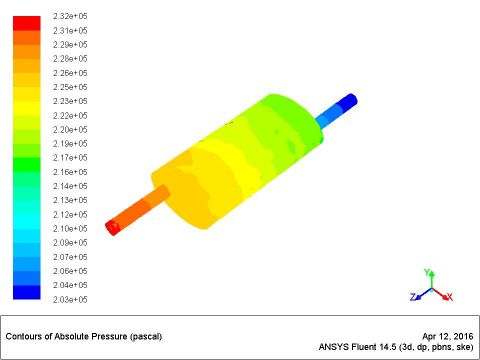Figure 7. Absolute pressure contour for non-perforated elliptical chamber muffler

## 8.pngFigure 8. Absolute pressure contour for perforated elliptical chamber muffler

Maximum value of absolute pressure is observed to be 2.32×105 Pa and 2.56×105 Pa for the non-perforated and perforated muffler model respectively from Figure 7 and 8 above.

## 9.png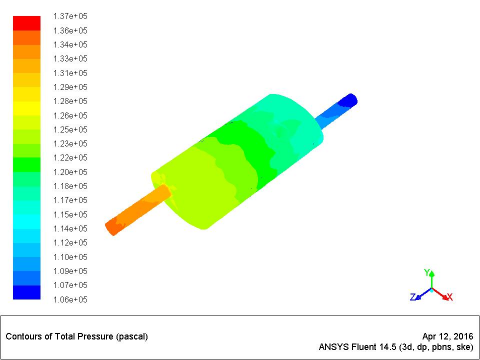Figure 9. Total pressure contour for non-perforated elliptical chamber muffler

## 10.png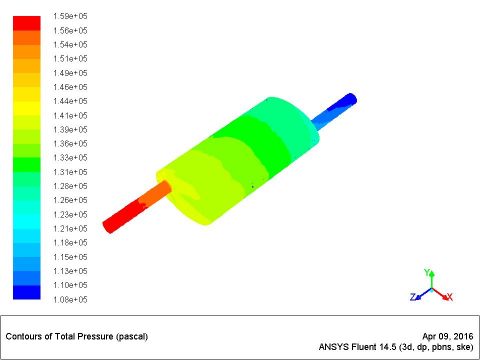Figure 10. Total pressure contour for perforated elliptical chamber muffler

Total pressure is seen to have maximum value of 1.37×105 and 1.59×105 Pa for the non-perforated and perforated muffler model respectively in Figure 9 and 10 above.

## 11.pngFigure 11. Contour of velocity vector for non-perforated elliptical chamber muffler

## 12.pngFigure 12. Contour of velocity vector for perforated elliptical chamber muffler

Exhaust gas flow through the muffler chamber is seen to have maximum values of 139.8 m/s and 154.2 m/s for the non-perforated and perforated model respectively as shown in the velocity vector contour in Figure 11 and 12 above. The arrows illustrate direction of gas flow through the muffler.

## 13.pngFigure 13. Turbulent intensity contour for non-perforated elliptical chamber muffler

## 14.png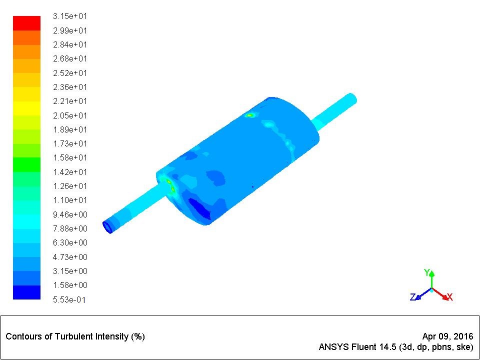Figure 14. Turbulent intensity contour for perforated elliptical chamber muffler

## 15.pngFigure 15. Acoustic power level contour for non-perforated elliptical chamber muffler

Turbulent intensity attains a maximum value of 59.2 % for exhaust gas flow through the non-perforated muffler model as seen from Figure 13. On the other hand, it is seen to attain a maximum value of 31.5 % for the perforated muffler model as shown in Figure 14. Higher turbulent intensity directly implies greater mechanical vibration inside the muffler chamber.

## 16.pngFigure 16. Acoustic power level contour for perforated elliptical chamber muffler

## 17.pngFigure 17. Surface acoustic power level contour for non-perforated elliptical chamber muffler

## 18.pngFigure 18. Surface acoustic power level contour for perforated elliptical chamber muffler

Acoustic power level contours are shown in Figure 15 and 16 above with maximum value of 166 dB and 149 dB for non-perforated and perforated muffler model respectively.

Surface acoustic power level acquires maximum values of 143 dB and 134 dB for the non-perforated and perforated model as seen in Figure 17 and 18 above.

5.2 Discussion

Results obtained from computational analysis of static pressure, velocity, turbulent intensity, acoustic power level and surface acoustic power level for both the non-perforated and perforated muffler models along with their percentage change is depicted in Table 1.

The computational analysis results point out increased values of static pressure along with velocity. Presence of perforation provides opposition to the flow of gases. To account for this opposition to flow, higher amount of pressure is generated inside the muffler chamber so as to allow exhaust gases to flow out. Reduction in area in the form of perforation leads to an increase in velocity to achieve constant volumetric flow rate.

On the other hand, turbulent intensity, acoustic power level and surface acoustic power level are seen to acquire decreased values. This is due to the fact that sound waves get reflected from the discontinuities and finally cancel each other out leading to reduced sound power and vibration within the chamber.

Table 1. CFD analysis results

 Parameter of analysis Non-perforated muffler model Perforated muffler model % change in perforated muffler Max. value Min.  value Max. value Min. value Static pressure (Pa) 1.31×105 1.01×105 1.55×105 0.985×105 15.5% increase Absolute pressure (Pa) 2.32×105 2.03×105 2.56×105 2.00×105 9.4% increase Total pressure (Pa) 1.37×105 1.06×105 1.59×105 1.08×105 13.8% increase Velocity vector (m/s) 139.8 0.0 154.2 0.0 9.3% increase Turbulent intensity (%) 59.2 0.442 31.5 0.553 46.8% decrease Acoustic power level (dB) 166 0 149 0 10.2% decrease Surface acoustic power level (dB) 143 0 134 0 6.3% decrease
6. Validation of Results

One of the major parameters for determination of muffler performance is transmission loss. It is the difference between the power incident at the inlet of a muffler and that transmitted downstream at the outlet and expressed in the unit of decibel. For better noise attenuation a higher value of transmission loss is desired. Mathematically, transmission loss is represented as follows:

$T.L=10{{\log }_{10}}\left| \frac{{{S}_{i}}{{p}_{{{i}^{2}}}}}{{{S}_{o}}{{p}_{{{o}^{2}}}}} \right|$ (4)

where,

Si and So are the cross-sectional areas of the inlet and outlet of the muffler.

pi and po are the acoustic pressure of the incident wave at the inlet of the muffler and transmitted wave at the outlet of the muffler respectively.

In the present case where the inlet and outlet of the muffler are of equal cross-sectional area the above formula can be represented in modified form as follows:

$T.L=20{{\log }_{10}}\left| \frac{{{p}_{i}}}{{{p}_{o}}} \right|$  (5)

Incorporating inlet and outlet acoustic pressure from CFD analysis results, transmission loss for non-perforated and perforated elliptical chamber muffler model is being calculated as shown in Table 2.

Table 2. Comparative calculations on transmission loss

 Non-perforated muffler model Perforated muffler model pi=130985.8 Pa; po=101264 Pa pi=154973.5 Pa; po=98476.5 Pa $T.L=20 \log _{10}\left|\frac{130985.8}{101264}\right|=$ 2.235$\approx 2 d B$ \begin{align} & T.L=20{{\log }_{10}}\left| \frac{154973.5}{98476.5} \right|= \\ & 3.938\approx 4dB \\ \end{align}

Comparison of the transmission loss calculation results yield a higher value of 4 decibel for the perforated muffler model as compared to 2 decibel for the non-perforated model thus validating its superior noise attenuation characteristic over the non-perforated muffler model. Transmission loss of an automobile muffler depends on the frequency of the engine and attains zero at resonant frequencies. Automobile frequency under normal circumstances vary between 50 to 3000 Hertz. For the MAHINDRA MAXIMO PLUS engine under study maximum attainable speed is 3600 rpm and working frequency is thus 60 Hertz.

Transfer matrix method (TMM) and experimental findings of Gerges et al.  and analytical findings of Denia et al.  using superposition method report a transmission loss of nearly 4 to 6 decibel at a frequency of 60 Hertz. The transmission loss obtained for the proposed muffler model is almost equal to this result thus validating its accuracy over the non-perforated muffler model.

Velocity vector contour for perforated muffler model depicts a maximum velocity of 154.2 m/s for flow of exhaust gases. This value is quite close to the one reported by Caradonna et al. . They reported a maximum velocity of 150 m/s by both experimental and analytical methods.

7. Conclusion

Static pressure, absolute pressure and total pressure distribution for both the non-perforated and perforated muffler models have been compared. Due to incorporation of discontinuities in the form of perforations, a higher amount of pressure distribution is generated across the proposed perforated muffler model. On the basis of the results of computational analysis, transmission loss for each muffler model has been calculated. The transmission loss is evidently seen to acquire a higher value for the perforated muffler model which validates its efficiency over the non-perforated muffler model in terms of sound attenuation. For an increase in static pressure by 15.5 %, there is an increase in transmission loss by 43.4 % for the proposed model of the elliptical chamber muffler. Along with pressure distribution there is an increase in maximum velocity by 9 % for the perforated design due to incorporation of area discontinuities or the perforations. This is again quite insignificant as opposed to the sound attenuation being achieved. Turbulent intensity, on the other hand, is found to be 46.8 % lesser for the perforated muffler model. At the same time, there is also a decrease in the acoustic power level and surface acoustic power level values for the proposed muffler model by 10.2 % and 6.3 % respectively.

As such, it can be concluded that the perforated muffler model definitely achieves better sound attenuation as compared to the non-perforated model at the cost of a small amount of increase in pressure which is well within the allowable range.

Nomenclature
 k turbulent kinetic energy, kg m2 s-2 u velocity, m s-1 G generation of kinetic energy g gravitational vector Pa pascal, N m-2 T.L Transmission Loss p Pressure

Greek symbols

 ε turbulent dissipation rate, m2 s3 µ dynamic viscosity, kg m-1.s-1 ρ density, kg m-3 b thermal expansion coefficient, K-1 δ differential operator

Suscripts

 i inlet o outlet T turbulence P constant pressure M Mach number
References

 Munjal, M.L. (1998). Analysis and design of mufflers. Journal of Sound and Vibration, 211(3): 425-433. https://doi.org/10.1006/jsvi.1997.1309

 Denia, F.D., Albelda, J., Fuenmayor, F.J. (2001). Acoustic behavior of elliptical chamber mufflers. Journal of Sound and Vibration, 241(3): 401-421. https://doi.org/10.1006/jsvi.2000.3289

 Gerges, S.N.Y., Jordan, R., Thieme, F.A., Coelho, J.L.B., Arenas, J.P. (2005). Muffler modeling by transfer matrix method and experimental verification. Journal of Brazilian Society of Mechanical Sciences and Engineering, 27(2): 132-140. https://doi.org/10.1590/S1678-58782005000200005

 Sohei, N., Tsuyoshi, N., Takashi, Y. (2006). Acoustic analysis of elliptical muffler chamber having a perforated pipe. Journal of Sound and Vibration, 297: 761-773. https://doi.org/10.1016/j.jsv.2006.04.026

 Caradonna, J., Schwartz, O., Francescantonio, P.D. (2010). Innovative computational aero-acoustic approach for automotive exhaust system. Proceedings of ISMA, pp. 611-624.

 Kang, Z., Zheng, S., Lian, X., Ji, Z. (2010). Study on the acoustic performance of perforated duct muffler. IEEE. https://doi.org/10.1109/MACE.2010.5535582

 Bhangale, K., Shivankar, R., Sharma, P.K. (2015). Vibration analysis for an automotive silencer for reduced incidence of failure. International Journal of Engineering and Technical Research, 3: 79-84.

 Wankhade, A.W., Bhattu, A.P. (2015). Optimization and experimental validation of elliptical reactive muffler with central inlet central outlet. International Journal of Engineering Research and Technology, 4(5): 1321-1328. https://doi.org/10.17577/IJERTV4IS051209

 Chatterjee, S. (2016). Computational fluid dynamic analysis of the exhaust gas flow through absorptive and reactive mufflers: some case studies. Proc IMechE Part D: Journal of Automobile Engineering, 231(11): 1568-1588. https://doi.org/10.1177/0954407016676230

 Baruah S., Chattejee S. (2018). Structural analysis for exhaust gas flow through an elliptical chamber muffler under static and dynamic loading condition. Advances in Modelling and Analysis B, 61(2): 92-98. https://doi.org/10.18280/ama_b.610207

 Launder, B.E., Spalding, D.B. (1972). Lectures in Mathematical Models of Turbulence. Academic Press, London, England. https://doi.org/10.1017/s0022112073222048

 Sarkar, S., Balakrishnan, L. (1991). Application of a Reynolds stress turbulence model to the compressible shear layer. AIAA Journal, 29(5): 743-749. https://doi.org/10.2514/3.10649

 Fomichev, D.V., Solonin, V.I. (2014). A CFD analysis of hydraulic characteristics of the rod bundles in the BREST-OD-300 wire-spaced fuel assemblies. International Conference on Nuclear Energy Science and Technology (ICNEST), At Zurich, pp. 692-697.